quadrilateral is a closed figure with four sides that are line segments.  The figures below are special types of quadrilaterals.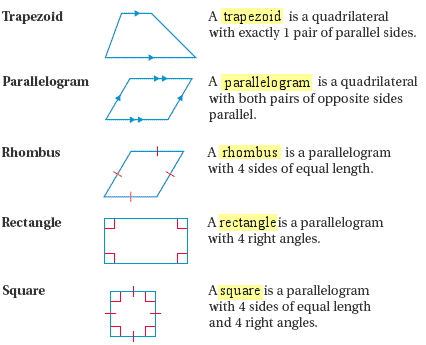Example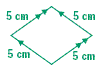Solution:

The quadrilateral is a parallelogram with 4 sides of equal length. So, it is a rhombus. It is also not a square because the angles are not right.

Finding Unknown Angle Measures in a Quadrilateral

The sum of the angle measures in a quadrilateral is 360 degrees.  If one angle measurement is unknown, algebra can be used to solve for the missing angle measure.

Example

Find the value of x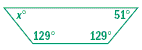Solution:

Recall that the sum of the angle measures in a quadrilateral is 360 degrees.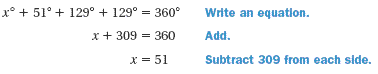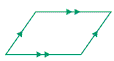2.  Complete each statement.

a.   A _?_ is a quadrilateral with both pairs of opposite sides parallel.

b.   A parallelogram with 4 right angles is a _?_.

c.   A _?_ is a quadrilateral with exactly 1 pair of parallel sides.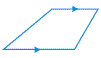4.  Which word can describe two adjacent sides of a rectangle?

A. vertical
B.
right
C.
acute
D. perpendicular

5.  Three angles in a quadrilateral are acute. Classify the fourth angle.

A. acute
B.
right
C.
obtuse
D. straight

6.  Is statement below is alwayssometimes, or never true?

a.  A rhombus is also a parallelogram.

b.  A rectangle is also a square.

c.  A square is also a parallelogram.

d.  A triangle is also a quadrilateral.

e.  A quadrilateral is also a rectangle.

7.  Find the value of x.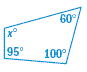1) Parallelogram

2)
a) Parallelogram
b) Rectangle
c) Trapezoid

3) Trapezoid

4) Perpendicular

5) Obtuse

6)
a) Always
b) Sometimes
c) Always
d) Never
e) Sometimes

7) 105